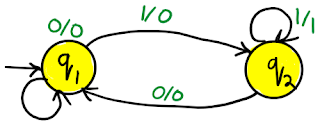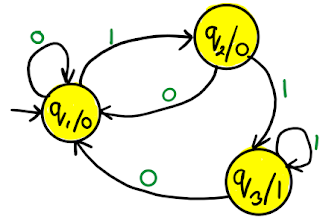# Define Mealy and Moore Machine

### RGPV 2010

#### 2. Moore machine

1. Mealy machine: Mealy machine is a six tuple machine.M = (Q, Σ, △, δ, λ, q0)

1. Q is finite set of states.
2. Σ is the input alphabet.
3. △ is the output alphabet.
4. δ is transition function which maps Q×∑ → Q.
5. ‘λ’ is the output function which maps Q×∑→ △.
6. q0 is the initial state.

Transition table for Mealy machine

Transition diagram for Mealy machine1. Moore machine: Moore machine is a six tuple machine.M = (Q, Σ, △, δ, λ, q0)

1. Q is finite set of states.
2. Σ is the input alphabet.
3. △ is the output alphabet.
4. δ is transition function which maps Q×∑ → Q.
5. ‘λ’ is the output function which maps Q → △.
6. q0 is the initial state.

Transition table for Moore machine

Transition diagram for Moore machine### Mealy machine vs Moore machine

Practice problems: